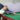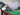Numbers speak truth

# Numbers speak truth

I am not a mathematician but I found this really interesting:

Assuming: A = 1 ; B = 2 ; C = 3 ; D = 4 —-> till Y = 25 ; Z = 26.

Then,

H+A+R+D+W+O+R+K =

8+1+18+4+23+15+18+11 = 98%

K+N+O+W+L+E+D+G+E =

11+14+15+23+12+5+4+7+5 =96%

L+U+C+K =

12+21+3+11 = 47%

None of them makes 100%.

Then what makes 100%?

Is it Money?

M+O+N+E+Y =

13+15+14+5+25 = 72%

L+E+A+D+E+R+S+H+I+P =

12+5+1+4+5+18+19+8+9+16 = 97%

However,

👉A+T+T+I+T+U+D+E = 1+20+20+9+20+21+4+5 = 100%👈

Numbers do speak sense!

#numbers

#GallaVichaar

## Recent Posts### I used to think ONLY working hard is the key to success.### We all deserve a better life.### Why do you want to work here?### Tracking incremental progress in business is often ignored.

#### Related Articles### I used to think ONLY working hard is the key to success.

I used to think ONLY working hard is the key to success....### We all deserve a better life.

An employee who resigned to join a competitor, asked me for a...### Why do you want to work here?

“Why do you want to work here?” Doesn’t this question sound ridiculous?...### Tracking incremental progress in business is often ignored.

Tracking incremental progress in business is often ignored. Consistency and small steps...